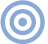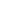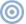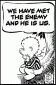# Reference

## NWAA Labs: Measurement Beyond The Atomic Level Shape and Absorption

Sidebar: NWAA's Ron Sauro on Shape and Absorption

Our conversation moved back and forth between absorption and diffusion. When, later, Ron attempted to separate the two, he produced a mini treatise on absorption. Lightly edited, that treatise follows.

Absorption is the most common treatment used in listening rooms. The measurement of absorption was first described by Wallace Sabine, who compared the reverberation times of a room with and without absorption. This difference was then converted to units of absorption using this formula:

A = 0.9210(V*d/c)
where
A = equivalent absorption area in m2,
V = volume of reverberation room in m,
c = speed of sound at ambient temperature and humidity in m/s,
and d = decay rate in dB/s.

Most of what we think we know about absorption is wrong! Absorption calculations in use today can err by as much as 85%. The biggest error concerns how important the area of absorption is in the calculation of how much absorption is needed.

Area is not the only factor that affects absorption. In standards used by either ISO (International Organization for Standardization) or ASTM (American Society for Testing and Materials), when absorbers are measured in reverberation rooms, the edges of the samples are covered with a material, such as painted wood or sheet metal, that is impervious to sound. The assembly is taped o the edge coverings and the coverings taped to the floor. This method removes the edge thickness from the calculation of the absorption coefficient. When this method of converting the amount of absorption into a constant was first proposed at an ASA meeting in 1927, it was questioned. But no one proposed an alternative method. The formula was a very simple:

a=(A1–A2)/S+a1
where
a = absorption coefficient of the specimen,
A1 = absorption of the empty reverberation room,
A2 =absorption of the room after the specimen is installed,
S = surface area of the specimen,
and a1 = absorption coefficient of the surface covered by the specimen.

Under the presupposition that all numbers resulting from this calculation will fall between 0 and 1, this theoretical absorption constant was then described as a mathematical coefficient.

Imagine the shocked looks when results exceeded 1. After the first attempt at an explanation failed, most agreed this was an effect of diffraction of the edge and of little consequence. The phenomenon was then renamed edge effect and invoked as the reason for numbers that did not add up mathematically. Even though no one could explain what edge effect was, they blamed it for the "bad" numbers.

As part of the process of measuring scattering coefficient, acousticians used the ISO 17497-2 method to determine the absorption of a circular sample of X area and convert it to an absorption coefficient. Since there is also a different standard ISO method, ISO 354, to measure the absorption of a rectangular sample of the same area, we at NWAA Labs thought it might be useful to compare the results of the two methods. When we did, the circular sample measured about 15%–20% less than the rectangular sample, even though they both covered the same area. We repeated the test many times and got the same results.

Next, we began a series of tests where we broke up the area into small segments in different configurations. Our results produced an ever-increasing absorption coefficient. When these tests were plotted on a graph as result vs area, the results were linear to a point where it was 99.9% certain that the ratio of linear edge length to area was the operative denominator in the coefficient calculation. Reports of these experiments in Institute of Noise Control Engineering and ASA papers exposed the ineffectiveness of the absorption coefficient as a true constant for calculations of absorption. Later papers showed that calculation to be off by as much as 85%.

You can change absorption by up to 80% just by changing the absorber's shape. If you take a piece of material, let's say 100 square feet, and you make it into a circle, it will provide the minimum amount of absorption. If you fashion it into a square, it will be just slightly more absorptive. But if you make it into a star shape, you get almost 100% more absorption out of the same piece of material.—Ron SauroARTICLE CONTENTSCOMMENTSThank you!

Other than the LS-360 turntable, did he mention which electronics [associated equipment] they prefer?

(Hidden joke disclaimer.)You captured it all perfectly. Best primer on the loudspeaker/room/listener interface I have ever read. Wow!Very nice article, I’ve had a fun over the years pointing out the room is the most important thing. Thanks for showcasing the people doing the heavy lifting.I've driven by many times and had no idea what was happening at Satsop. Very interesting article, even without the local angle.

X